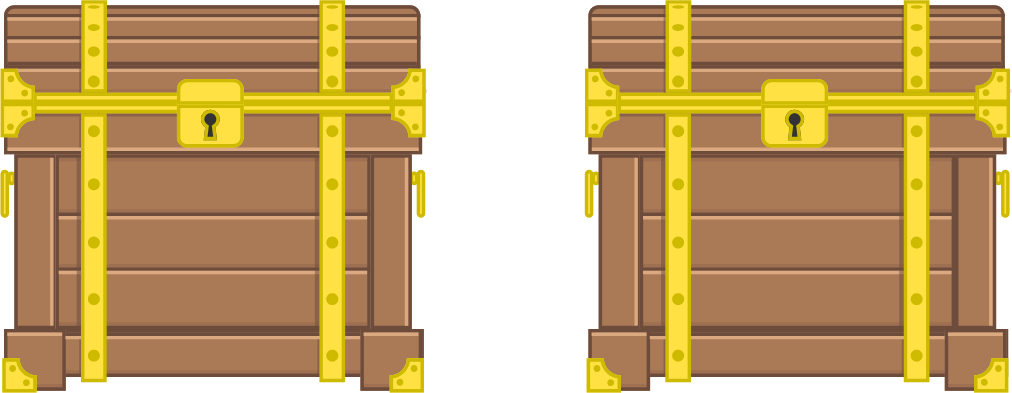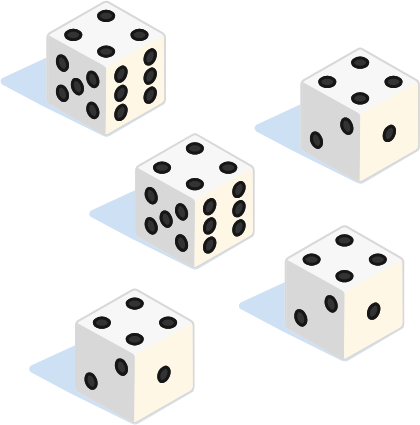# Problems of the Week

Contribute a problemThere are two chests. One will open if you say a true statement and the other will open if you say a false statement, but you don't know which is which!

You also know that one contains treasure and the other will release a deadly gas, but again, you don't know which.

Is it possible to make a statement that will cause only the chest with treasure to open?

Hint: Each statement is either true or false. It is not possible for a statement to be a paradox!

$n$ students are arranged in a line such that each student is sitting next to their good friends, and each student has no other good friends other than the students they are sitting next to.

If a teacher randomly reallocates their seats, what is the probability $P_n$ that none of these $n$ students sit next to their good friends?

Submit your answer as the limit $\displaystyle \lim_{n\to\infty} P_n.$

Inspiration

The Friedmann equation, derived from Einstein's theory of general relativity, describes the expansion of the Universe after the Big Bang. The equation is, in a slightly simplified form, $\left( \frac{\dot a}{a} \right)^2 = H_0^2 \left( \frac{1-\Omega}{a^3}+\Omega \right),$ where $\Omega$ is the fraction of dark energy in the Universe, $H_0$ is the Hubble constant today, and $a(t)$ is a dimensionless "size factor" selected so that its value today is $a=1$ $\big($for example, $a(t)=R(t)/R_0$, the distance $R$ between Earth and a distant galaxy at time $t$ divided by the current distance$\big).$

Based on the solution of this equation, what was the scale factor $a$ when the Universe was half of the age today?

Notes: Use $\Omega=0.68$. The quantity $1-\Omega=0.32$ represents the fraction of matter (regular and dark) in the Universe. The Hubble constant is $H_0=\dfrac{1}{14\times 10^9\text{ years}}$. We neglected the energy contained by radiation and assumed that the Universe is flat, i.e. the matter and energy fractions add up to 1.I play a round of the game "Yahtzee!", and my goal is to obtain the namesake combination: 5 dice all showing the same number.

What is the probability that I will be able to accomplish this?

The probability can be expressed as $\frac{a}{b},$ where $a$ and $b$ are coprime positive integers. Enter your answer as $a + b$.

Details and Assumptions:

• Each round of Yahtzee consists of up to 3 tosses. In the first toss, you roll all 5 dice.
• In the second toss, you may roll some or all of the 5 dice.
• In the third toss, you may roll some or all of the 5 dice.
• The probability to obtain a "Yahtzee" assumes that you follow the optimal strategy.
• It would be acceptable to use a calculator or computer software to help with computations.The surface of a soccer ball is covered with pentagons and hexagons in such a way that one pentagon and two hexagons meet at each vertex.

Now, more mathematically, let's assume that a soccer ball is a truncated icosahedron with 12 identical regular pentagons and 20 identical regular hexagons.

The fraction of the pentagonal area on the surface of the polyhedral soccer ball can be expressed as $F = \frac \varphi {\varphi + \sqrt{a - b\varphi^2}},$ where $\varphi = \frac {1+\sqrt 5}2$ is the golden ratio, and $a,b$ are integers. What is $a-b?$

×